Courses

# Differential and Integral Calculus - 2 Notes | EduRev

## CA Foundation: Differential and Integral Calculus - 2 Notes | EduRev

The document Differential and Integral Calculus - 2 Notes | EduRev is a part of the CA Foundation Course Quantitative Aptitude for CA CPT.
All you need of CA Foundation at this link: CA Foundation

3 The Definite Integrals and the Fundamental Theorem

3.1 Riemann sums

Definition 1: Suppose f x) is nite-valued and piecewise continuous on [a; b]. Let P = {x0 = a; x1; x2 ; : : : ; xn = b} be a partition of [a; b] into n subintervals Ii = [xi-1 ; xi] of width Δxi = xi  - xi-1 , i = 1; 2; : : : ; n. (Note in a special case, we can partition it into subintervals of equal width: Δxi = xi  - xi-1  = w = (b - a)/n for all i). Let x*i be a point in Ii such that xi  - xi-1 ≤ x*i ≤ x(Here are some special ways to choose x*: (i) left endpoint rule x*i= xi-1  , and (ii) the right endpoint rule x*i = xi).

The Riemann sums of f (x) on the interval [a; b] are defined by: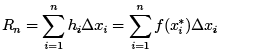which approximate the area between f (x) and the x-axis by the sum of the areas of n thin rectangles (see figure).

Example 1: Approximate the area under the curve of y = ex on [0; 1] by 10 rectangles of equal width using the left endpoint rule.

Solution: We are actually calculating the Riemann sum R10 . The width of each rectangle is Δxi = w = 1/10 = 0:1 (for all i). The left endpoint of each subinterval is xi = xi - 1 = (i -1)/10. Thus,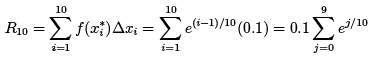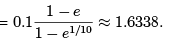3.2 The definite integral

Definition 1: Suppose f (x) is finite-valued and piecewise continuous on [a; b]. Let P = {x0 = a; x1 ; x2....... x= b} be a partition of [a; b] with a length defined by |p| = M ax1 ≤ i ≤ n { Δxi( (i.e. the longest of all subintervals). The definite integral of f (x) on [a; b] is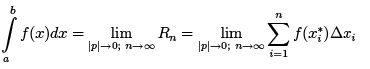where the symbol means "to integrate", the function f (x) to be integrated is called the integrand, x is called the integration variable which is a"dummy" variable, a and b are,respectively, the lower (or the starting) limit and the upper (or the ending) limit of the integral.
Thus,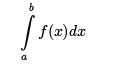means integrate the function f (x) starting from x = a and ending at x = b.

Example 2: Calculate the de nite integral of f(x) = x2 on [0:2].

Solution 2: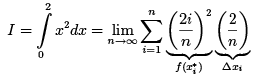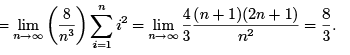Important remarks on the relation between an area and a de nite integral:

• An area, de ned as the physical measure of the size of a 2D domain, is always nonnegative.
• The value of a definite integral, sometimes also referred to as an "area", can be both positive and negative.
• This is because: a definite integral = the limit of Riemann sums. But Riemann sums are defined as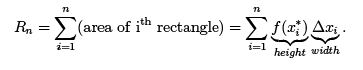• Note that both the hieght f (xi* ) and the width Δxican be negative implying that Rn can have either signs.

5 Differentials

De nition: The differential, dF , of any differentiable function F is an in nitely small increment or change in the value of F .

Remark: dF is measured in the same units as F itself.

Example: If x is the position of a moving body measured in units of m (meters), then its differential, dx, is also in units of m. dx is an in nitely small increment/change in the position x.

Example: If t is time measured in units of s (seconds), then its differential, dt, is also in units of s. dt is an in nitely small increment/change in time t.

Example: If A is area measured in units of m2 (square meters), then its differential, dA, also is in units of m2 . dA is an in nitely small increment/change in area A.

Example: If V is volume measured in units of m(cubic meters), then its differential, dV , also is in units of m3 . dV is an in nitely small increment/change in volume V .

Example: If v is velocity measured in units of m=s (meters per second), then its differential, dv, also is in units of m=s. dv is an in nitely small increment/change in velocity v.

Example: If C is the concentration of a biomolecule in our body fluid measured in units of M (1 M = 1 molar = 1 mole=litre, where 1 mole is about 6:023 x 1023 molecules), then its differential, dC , also is in units of M . dC is an in nitely small increment/change in the concentration C .

Example: If m is the mass of a rocket measured in units of kg (kilograms), then its differential, dm, also is in units of kg. dm is an in nitely small increment/change in the mass m.

Example: If F (h) is the culmulative probability of nding a man in Canada whose height is smaller than h (meters), then dF is an in nitely small increment in the probability.

De nition: The derivative of a function F with respect to another function x is de ned as the quotient between their differentials: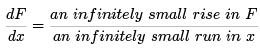Example: Velocity as the rate of change in position x with respect to time t can be expressed as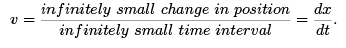Remark: Many physical laws are correct only when expressed in terms of differentials.

Example: The formula (distance) = (velocity) x (time interval) is true either when the velocity is a constant or in terms of differentials, i.e., (an inf initely small distance) = (velocity) x (an inf initely short time interval). This is because in an in nitely short time interval, the velocity can be considered a constant. Thus,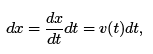which is nothing new but v(t) = dx=dt.

Example: The formula (work) = (force) x (distance) is true either when the force is a constant or in terms of differentials, i.e., (an inf initely small work) = (force) x (an infinitely small distance). This is because in an in nitely small distance, the force can be considered a constant. Thus,which is nothing new but v(t) = dx=dt.

Example: The formula (work) = (force) x (distance) is true either when the force is a constant or in terms of differentials, i.e., (an inf initely small work) = (force) x (an infinitely small distance). This is because in an in nitely small distance, the force can be considered a constant. Thus,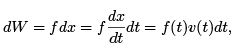which simply implies: (1) f = dW/dx, i.e., force f is nothing but the rate of change in work W with respect to distance x; (2) dW/dt = f (t)v(t), i.e., the rate of change in work W with respect to time t is equal to the product between f (t) and v(t).

Example: The formula (mass) = (density)x (volume) is true either when the density is constant or in terms of differentials, i.e., (an inf initely small mass) = (density)x(volume of an inf initely small volume). This is because in an in nitely small piece of volume, the density can be considered a constant. Thus,

dm = ρdV;

which implies that ρ = dm/dV, i.e., density is nothing but the rate of change in mass with respect to volume.

6 The Chain Rule in Terms of Differentials

When we differentiate a composite function, we need to use the Chain Rule. For example, f (x) = ex2 is a composite function. This is because f is not an exponential function of x but it is an exponential function of u = x2 which is itself a power function of x. Thus,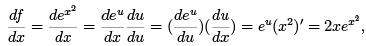where a substitution u = x was used to change f (x) into a true exponential function f (u).
Therefore, the Chain Rule can be simply interpreted as the quotient between df and dx is equal to the quotient between df and du multiplied by the quotient between du and dx. Or simply, divide df =dx by du and then multiply it by du.

However, if we simply regard x2 as a function different from x, the actual substitution u = x2 becomes unnecessary. Instead, the above derivative can be expressed as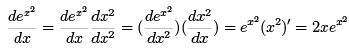Generally, if f = f (g(x)) is a differentiable function of g and g is a differentiable function of x, then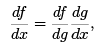where df , dg, and dx are, respectively, the differentials of the functions f , g, and x.

Example: Calculate df /dx for f (x) = sin(ln(x2 + ex)).

Solution: f is a composite function of another composite function!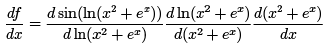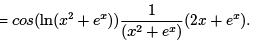7 The Product Rule in Terms of Differentials

The Product Rule says if both u = u(x) and v = v(x) are differentiable functions of x, then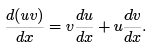Multiply both sides by the differential dx, we obtain

d(uv) = vdu + udv;

which is the Product Rule in terms of differentials.

Example: Let u = x2 and v = esin(x2), then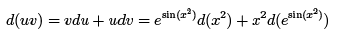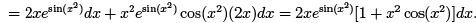8 Other Properties of Differentials

1. For any differentiable function F(x), dF = F'(x)dx (Recall that F'(x) = dF/dx!).

2. For any constant C , dC = 0.

3. for any constant C and differentiable function F (x), d(CF ) = C dF = C F'(x)dx.

4. For any differentiable functions u and v, d(u +- v) = du +- dv.

The document Differential and Integral Calculus - 2 Notes | EduRev is a part of the CA Foundation Course Quantitative Aptitude for CA CPT.
All you need of CA Foundation at this link: CA FoundationUse Code STAYHOME200 and get INR 200 additional OFF Use Coupon Code
All Tests, Videos & Notes of CA Foundation: CA Foundation## Quantitative Aptitude for CA CPT

66 docs|68 tests

Track your progress, build streaks, highlight & save important lessons and more!

,

,

,

,

,

,

,

,

,

,

,

,

,

,

,

,

,

,

,

,

,

;